The MEANS Procedure

# Concepts: MEANS Procedure

### Using TYPES and WAYS Statements

The TYPES statement controls which of the available class variables PROC MEANS uses to subgroup the data. The unique combinations of these active class variable values that occur together in any single observation of the input data set determine the data subgroups. Each subgroup that PROC MEANS generates for a given type is called a level of that type. Note that for all types, the inactive class variables can still affect the total observation count of the rejection of observations with missing values.

When you use a WAYS statement, PROC MEANS generates types that correspond to every possible unique combination of n class variables chosen from the complete set of class variables. For example

```proc means;
class a b c d e;
ways 2 3;
run;```

is equivalent to

```proc means;
class a b c d e;
types a*b a*c a*d a*e b*c b*d b*e c*d c*e d*e
a*b*c a*b*d a*b*e a*c*d a*c*e a*d*e
b*c*d b*c*e c*d*e;
run;```

If you omit the TYPES statement and the WAYS statement, then PROC MEANS uses all class variables to subgroup the data (the NWAY type) for displayed output and computes all types (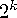) for the output data set.

### Ordering the Class Values

PROC MEANS determines the order of each class variable in any type by examining the order of that class variable in the corresponding one-way type. You see the effect of this behavior in the options ORDER=DATA or ORDER=FREQ. When PROC MEANS subdivides the input data set into subsets, the classification process does not apply the options ORDER=DATA or ORDER=FREQ independently for each subgroup. Instead, one frequency and data order is established for all output based on a nonsubdivided view of the entire data set. For example, consider the following statements:

```data pets;
input Pet \$ Gender \$;
datalines;
dog  m
dog  f
dog  f
dog  f
cat  m
cat  m
cat  f
;

proc means data=pets order=freq;
class pet gender;
run;```

The statements produce this output.

```                                 The SAS System                                1

The MEANS Procedure

N
Pet         Gender      Obs
---------------------------
dog         f             3

m             1

cat         f             1

m             2
---------------------------
```

In the example, PROC MEANS does not list male cats before female cats. Instead, it determines the order of gender for all types over the entire data set. PROC MEANS found more observations for female pets (f=4, m=3).

PROC MEANS uses the same memory allocation scheme across all operating environments. When class variables are involved, PROC MEANS must keep a copy of each unique value of each class variable in memory. You can estimate the memory requirements to group the class variable by calculating
where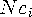is the number of unique values for the class variable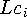is the combined unformatted and formatted length of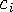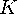is some constant on the order of 32 bytes (64 for 64-bit architectures).
When you use the GROUPINTERNAL option in the CLASS statement,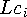is simply the unformatted length of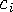.

Each unique combination of class variables,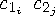for a given type forms a level in that type (see TYPES Statement). You can estimate the maximum potential space requirements for all levels of a given type, when all combinations actually exist in the data (a complete type), by calculating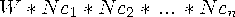where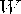is a constant based on the number of variables analyzed and the number of statistics calculated (unless you request QMETHOD=OS to compute the quantiles).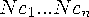are the number of unique levels for the active class variables of the given type.
Clearly, the memory requirements of the levels overwhelm the levels of the class variables. For this reason, PROC MEANS can open one or more utility files and write the levels of one or more types to disk. These types are either the primary types that PROC MEANS built during the input data scan or the derived types.

If PROC MEANS must write partially complete primary types to disk while it processes input data, then one or more merge passes can be required to combine type levels in memory with the levels on disk. In addition, if you use an order other than DATA for any class variable, then PROC MEANS groups the completed types on disk. For this reason, the peak disk space requirements can be more than twice the memory requirements for a given type.

When PROC MEANS uses a temporary work file, you will receive the following note in the SAS log:

```Processing on disk occurred during summarization.
Peak disk usage was approximately nnn Mbytes.
Adjusting SUMSIZE may improve performance.```

In most cases processing ends normally.

When you specify class variables in a CLASS statement, the amount of data-dependent memory that PROC MEANS uses before it writes to a utility file is controlled by the SAS system option and PROC option SUMSIZE=. Like the system option SORTSIZE=, SUMSIZE= sets the memory threshold where disk-based operations begin. For best results, set SUMSIZE= to less than the amount of real memory that is likely to be available for the task. For efficiency reasons, PROC MEANS can internally round up the value of SUMSIZE=. SUMSIZE= has no effect unless you specify class variables.

As an alternative, you can set the SAS system option REALMEMSIZE= in the same way that you would set SUMSIZE=. The value of REALMEMSIZE= indicates the amount of real (as opposed to virtual) memory that SAS can expect to allocate. PROC MEANS determines how much data-dependent memory to use before writing to utility files by calculating the lesser of these two values:

• the value of REALMEMSIZE=

• 0.8*(M-U), where M is the value of MEMSIZE= and U is the amount of memory that is already in use.

Operating Environment Information:   The REALMEMSIZE= SAS system option is not available in all operating environments. For details, see the SAS Companion for your operating environment.If PROC MEANS reports that there is insufficient memory, then increase SUMSIZE= (or REALMEMSIZE=). A SUMSIZE= (or REALMEMSIZE=) value that is greater than MEMSIZE= will have no effect. Therefore, you might also need to increase MEMSIZE=. If PROC MEANS reports insufficient disk space, then increase the WORK space allocation. See the SAS documentation for your operating environment for more information on how to adjust your computation resource parameters.

Another way to enhance performance is by carefully applying the TYPES or WAYS statement, limiting the computations to only those combinations of class variables that you are interested in. In particular, significant resource savings can be achieved by not requesting the combination of all class variables.Previous Page | Next Page | Top of Page